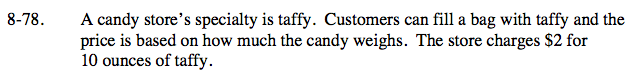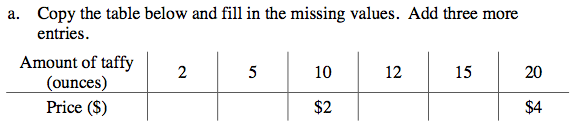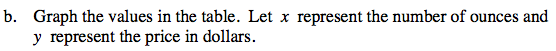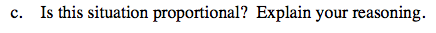Home > CC2 > Chapter 8 > Lesson 8.3.2 > Problem8-78

8-78.
1. A candy store’s specialty is taffy. Customers can fill a bag with taffy and the price is based on how much the candy weighs. The store charges $2 for 10 ounces of taffy. 8-78 HW eTool (Desmos). Homework Help ✎ 1. Copy the table below and fill in the missing values. Add three more entries.  Amount of taffy (ounces) 2 5 10 12 15 20 Price ($) $2$4
2. Graph the values in the table. Let x represent the number of ounces and y represent the price in dollars.

3. Is this situation proportional? Explain your reasoning.Try writing a proportion equation.

$\frac{10\text{ ounces}}{2\text{ dollars}}=\frac{2\text{ ounces}}{x\text{ dollars}}$

Solve for x.Does the price per ounce stay the same no matter how many ounces of taffy is bought?

Notice that the graph makes a straight line and intersects (0,0).

Complete the table in the eTool below to graph the points.
Click the link at right for the full version of the eTool: CC2 8-78 HW eTool